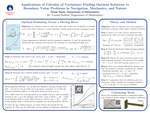## Stander Symposium Projects

#### Presenter(s)

Presentation: 10:45 a.m.-12:00 p.m., Kennedy Union Ballroom

#### Description

Calculus of variations is a branch of mathematics that deals with the optimization of functionals, which are functions that assign a scalar value to an input function. A functional could be a measurement of quantities such as time, cost, or energy, while also under boundary value constraints such as physical limitations. To find optimal functions of the functional, one uses the Euler-Lagrange equation to derive a solution function and verifies it satisfies the Legendre condition to prove the solution is the optimal one. To illustrate the applicability and effectiveness of the technique, this project investigates multiple boundary value problems, such as the quickest path to cross a moving river, the maximum deflection of materials under stress, and deriving (naturally occurring) optimal curves. These examples demonstrate how calculus of variations can be used to both solve for optimal solutions and derive the equations that are already represented in physical systems.

4-19-2023

Capstone Project

Youssef Raffoul

Mathematics

#### Keywords

Stander Symposium, College of Arts and Sciences

#### Institutional Learning Goals

ScholarshipCOinS